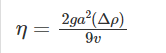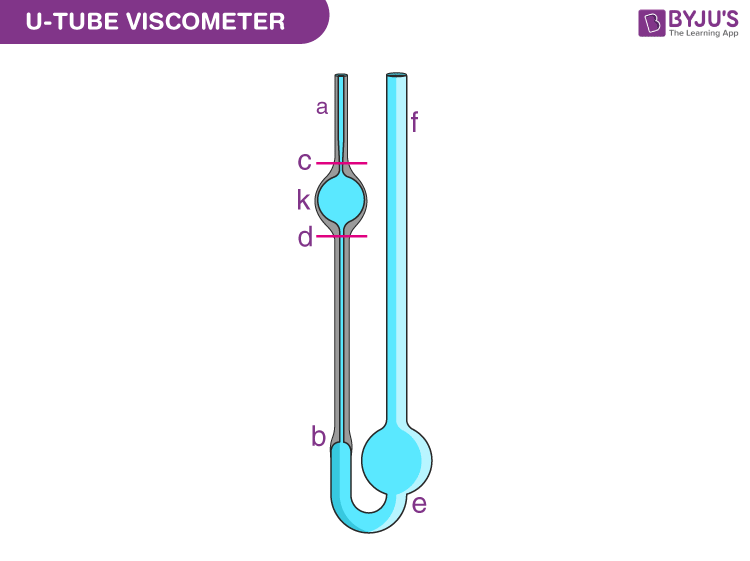Jet Set Go! All about Aeroplanes Jet Set Go! All about Aeroplanes

# Viscosity Questions

The resistance to the flow of a fluid, as well as a gas’s resistance to flow, change shape, or movement, is known as viscosity. The reverse of viscosity is fluidity, which measures the ease with which a liquid flows, whereas viscous liquids are those that are “sluggish” and high in viscosity, such as motor oil or honey.

It’s also important to remember that temperature affects the viscosity of liquids and gases in different ways, meaning that the viscosity of a liquid drops rapidly when heated, whereas gases move more slowly. What is the reason for this? The average speed of molecules in a liquid increase as the temperature rises, and as a result, they spend less time with their “neighbours.” As a result, as the temperature rises, the average intermolecular forces drop, allowing molecules to interact without being “weighed down.”

 Definition: Viscosity is the resistance of a fluid (liquid or gas) to changing shape or moving neighbouring sections relative to one another. The term “viscosity” represents the resistance to flow. Fluidity is characterized as the reciprocal of viscosity, which is a measure of flow ease. Molasses, for example, has a viscosity that is higher than water.

## Viscosity Chemistry Questions with Solutions

Q1: The viscosity of liquid

A. Increases with increase in temperature

B. Decreases with increase in temperature

C. Decreases with decrease in temperature

D. Remains constant regardless of any change in the temperature

Answer: B. Decreases with increase in temperature

Explanation: The viscosity of the fluid decreases as the temperature increases. This is due to a reduction in intermolecular forces.

Q2: What is the relation between the viscosities of blood and water?

A. Both are equal.

B. Water is more viscous than blood.

C. They cannot be compared.

D. Blood is more viscous than water.

Answer: D. Blood is more viscous than water.

Q3: The factor on which viscosity depends is __________

A. Area of contact of two adjacent layers

B. Velocity difference between two adjacent layers

C. The distance between two adjacent layers

D. All of the mentioned

Answer: D. All of the mentioned

Explanation: The area of contact between two adjacent layers, the velocity difference between two adjacent layers, and the distance between two adjacent layers are all factors that influence viscosity.

Q4: What is the SI unit of viscosity?

A. Poiseiulle

B. Pascal/second

C. Newton-Second

D. Newton/Second

Q5: What is the dimension of viscosity?

A. MLT2

B. M1L-1T

C. ML-1T-1

D. ML-2T1

Explanation: The dimension of viscosity is ML-1T-1. It’s unit is m2/s or stokes.

Q6: Select the incorrect statement from the following option.

A. Viscosity helps in the selection of good lubricating oil

B. Light oils have low densities and easy flowability

C. Heavy oils are used on parts moving with high speed

D. Light oils do not impose much drag on high-speed parts

Answer: C. Heavy oils are used on parts moving with high speed

Explanation: On parts moving slowly under heavy loads, heavy oils are employed. All of the other possibilities are correct. Light oils have low densities, smooth permeability, and do not impose much drag on high-speed parts, hence viscosity aids in the selection of a good lubricating oil.

Q7: Give the formula of Viscosity.

The ratio of shearing stress to velocity gradient in a fluid is used to calculate viscosity. The viscosity of a fluid can be determined by dropping a sphere into it and using the following formula:Where ∆ρ is the density difference between the fluid and the sphere being tested, ‘a’ is the sphere’s radius, ‘g’ is gravity’s acceleration, and ‘v’ is the sphere’s velocity.

Q8: What are the types of Viscosity?

The viscosity of a fluid is a measurement of its hindrance to flow. There are two methods for determining the viscosity of a fluid:

• Dynamic Viscosity (Absolute Viscosity)
• Kinematic Viscosity

One method to measure the fluid’s resistance to flow when a force is applied to it is Dynamic Viscosity. The other method is to measure the fluid’s resistive flow under gravity’s weight. Kinematic viscosity is the term given to this measure of fluid viscosity.

Many people don’t understand the difference between the two viscosity measurements and believe they’re the same thing. They are, in fact, significantly different. Kinematic viscosity is much more beneficial than absolute or dynamic viscosity in a few circumstances.

Q9: What are the forces which are responsible for the viscosity of a liquid? Why is glycerol more viscous than water?

The forces that cause liquid viscosity are as follows:

• Hydrogen bonding
• van der Waals torches.

Glycerol has a higher viscosity than water because it has more hydrogen bonding in its molecules due to the pressure of three-Oil groups in it, whereas water molecules have less hydrogen bonding.

Q10: Describe an U-Tube Viscometer.

Glass capillary viscometers and Ostwald viscometers are other names for U-tube viscometers.

Two reservoir bulbs and a capillary tube make up a viscometer. The capillary, a vertical segment of a very narrow bore, is located in one of the U’s arms. As indicated in the illustration, there is a light above it, and another bulb lower down on the other arm.The upper bulb suctions the liquid, which is then forced to flow down via the capillary into the lower bulb. A known volume is indicated by two markers (one above and one below the upper bulb). The kinematic viscosity is proportional to the time it takes for the liquid to move between these points.

A conversion factor is included with most commercial units. The test liquid’s time to flow between two places is calculated. The kinematic viscosity is calculated by multiplying the time measured by the factor of the viscometer.

Q11: Explain Coefficient of viscosity.

The liquid layer in contact with fixed surface AB does not move when liquid flows across a flat surface. The upper and upper layers advance at a faster rate. A backward dragging force F operates tangentially on each layer due to relative motion.

Hence, coefficient of viscosity might be defined as (or is equal to) the tangential force needed to maintain a unit velocity gradient between two layers each of unit area.

Unit of the coefficient of viscosity is measured in C.G.S. units (P).

Q12: Why liquids have a definite volume, but no definite shape?

It’s because intermolecular forces in liquids are strong enough to hold the molecules together, but not strong enough to fix them into definite or concrete locations like they are in solids. As a result, they are fluid but lack a defined shape.

Q13: How does temperature affect the viscosity of a fluid?

Because viscosity is primarily concerned with how molecules move and are bound to one another, temperature is inversely related to it. High temperature induces the molecules to move faster, making fluid less viscous.

Pressure, on the other hand, is related to viscosity: increased pressure decreases molecular mobility. LPG, for example, has a higher viscosity inside the tank due to the higher pressure, which turns the gas into a liquid.

Both the temperature and the pressure are kept at standard settings based on the 1982 IUPAC conventions in order to obtain an accurate and uniform measurement of viscosity:

• 273.15 K (0 °C) temperature
• 100 kPa (roughly 1 atm) pressure

This means viscosities of various fluids can be accurately compared.

Q14: What is oil viscosity?

The viscosity of engine oil is the ease with which it pours at a given temperature. Thin oils have a lower viscosity than thicker oils with a higher viscosity, therefore they pour more easily at low temperatures. Thin oils help engines start faster in cold weather by reducing friction in the engine.

Q15: A square plate 0.1 m side moves parallel to second plate with a velocity of 0.1 ms−1, both plates being immersed in water. If the viscous force is 0.002 N and the coefficient of viscosity 0.001 poise, what is the distance between the plates?

Given data,

Area = a2 = 1 x 0.1 = 0.1 m2

η = 0.01 poise

⇒ η = 0.01/10 = 0.001 deca poise

dv = 0.1 m/s

F = 0.002 N

We know that,

As

$$\begin{array}{l}F = \eta A\frac{dv}{dx}\end{array}$$

Or,

$$\begin{array}{l}dx = \eta \frac{Adv}{F}\end{array}$$
$$\begin{array}{l}=\frac{0.001 x (0.1)^{2} x 0.1}{0.002}\end{array}$$

= 0.0005 m

## Practise Questions on Viscosity

Q1: The highest viscosity among the following is of

A. water

B. air

C. blood

D. honey

E. None of the above / More than one of the above

Q2: How does viscosity gets affected on increasing the pressure?

A. Viscosity increases

B. Viscosity decreases

C. Viscosity does not get affected by the pressure

D. None of the mentioned

Q3: What do the critical temperature, critical pressure, and critical volume for a gas stand for?

Q4: Is there any effect of the nature of a liquid and temperature on the surface tension of a liquid?

Q5: What do you understand from surface tension?

Click the PDF to check the answers for Practice Questions.

## Viscosity: States of Matter Class 11 Chemistry